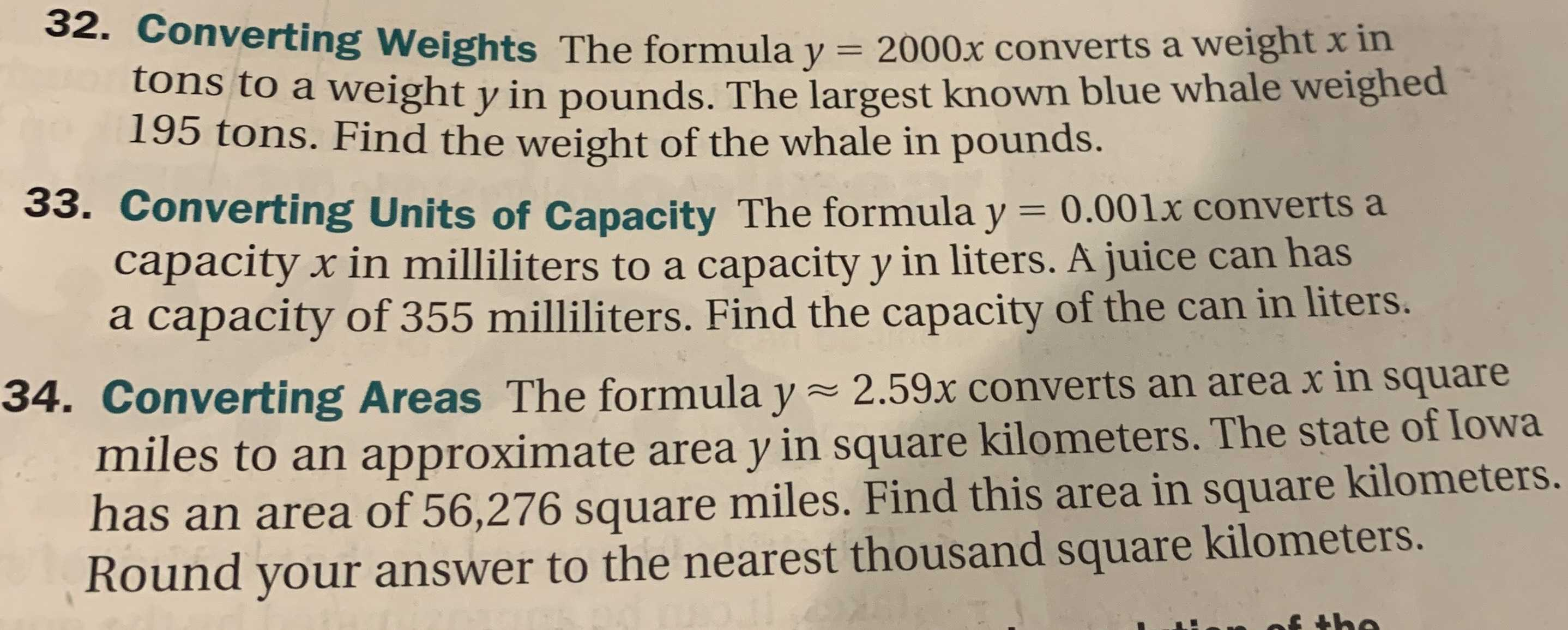### ¿Todavía tienes preguntas de matemáticas?

Pregunte a nuestros tutores expertos
Algebra
Pregunta32. Converting Weights The formula $$y = 2000 x$$ converts a weight $$x$$ in tons to a weight $$y$$ in pounds. The largest known blue whale weighed $$195$$ tons. Find the weight of the whale in pounds. 33. Converting Units of Capacity The formula $$y = 0.001 x$$ converts a capacity $$x$$ in milliliters to a capacity $$y$$ in liters. A juice can has a capacity of $$355$$ milliliters. Find the capacity of the can in liters. 34. Converting Areas The formula $$y \approx 2.59 x$$ converts an area $$x$$ in square miles to an approximate area $$y$$ in square kilometers. The state of Iowa has an area of $$56,276$$ square miles. Find this area in square kilometers. Round your answer to the nearest thousand square kilometers.